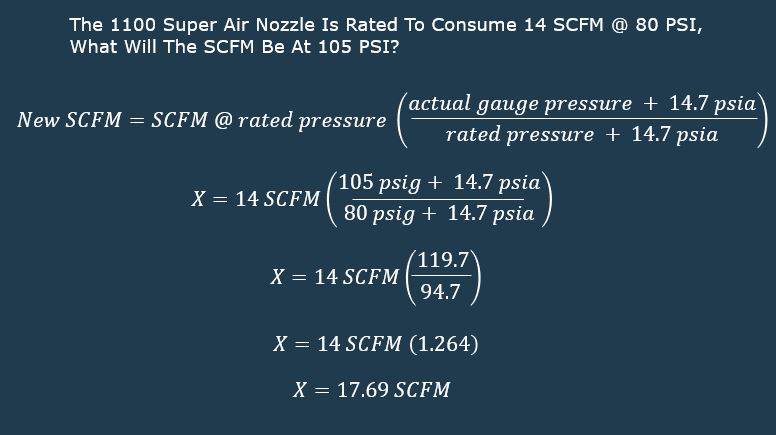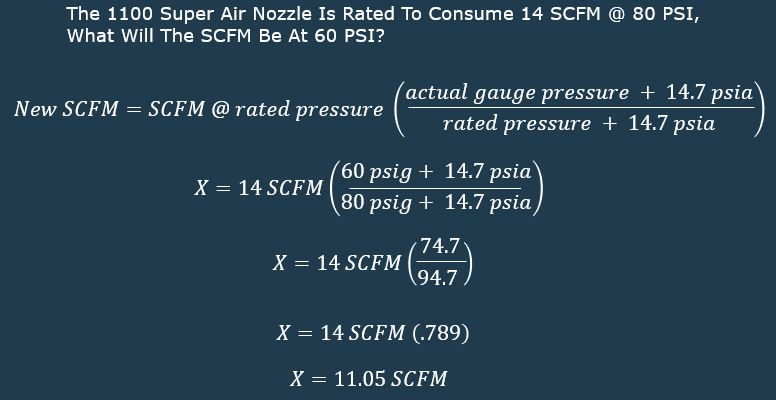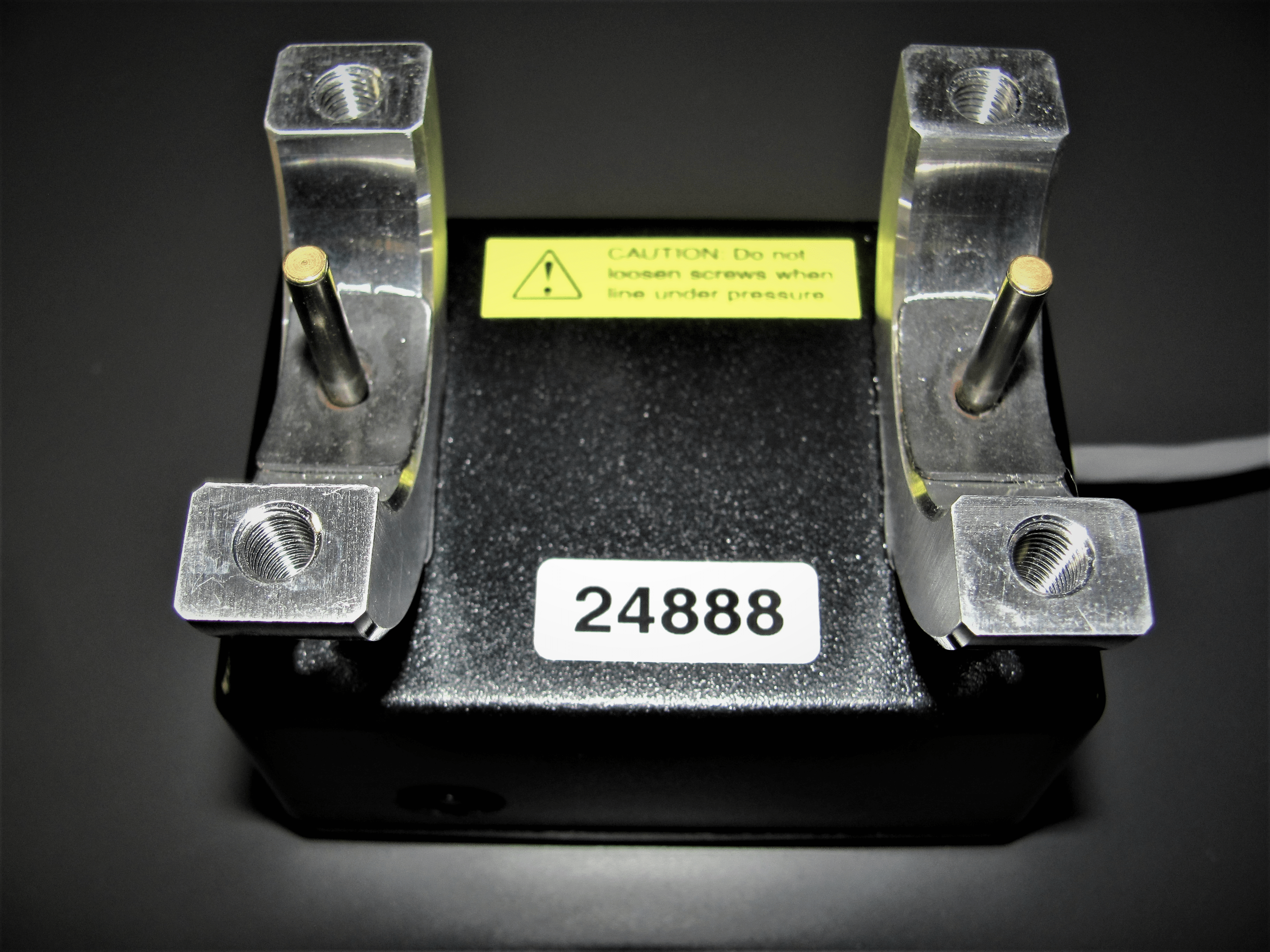## ICFM, SCFM, ACFM, CFM What does it all mean!

A common question we get asked is “What does SCFM mean?” Most people are aware of CFM but the “S” in front seems to be less known about! Well strap on your seat belt, we are about to go into a compressed air worm hole all about volumetric flow rates!

Here at EXAIR we rate all of our products air consumption in SCFM at a given supply pressure. CFM stands for Cubic Feet per Minute, but one definition will not satisfy the conditions that will be experienced in many applications by a number of variables  (altitude, temperature, pressure, etc.). Air by nature is a compressible fluid. The properties of this fluid are constantly changing due to the ambient conditions of the surrounding environment.

This makes it difficult to describe the volumetric flow rate of the compressed air. Imagine you have a cubic foot of air, at standard conditions (14.696 psia, 60°F, 0% Relative Humidity), right in front of you. Then, you take that same cubic foot, pressurize it to 100 psig and place it inside of a pipe. You still have one cubic foot, but it is taking up significantly less volume. You have probably heard the terms SCFMACFM, and ICFM when used to define the total capacity of a compressor system. Understanding these terms, and using them correctly, will allow you to properly size your system and understand your total compressed air consumption.

SCFM is used as a reference to the standard conditions for flow rate. This term is used to create an “apples to apples” comparison when discussing compressed air volume as the conditions will change. EXAIR publishes the consumption of all products in SCFM for this reason. You will always notice that an inlet pressure is specified as well. This allows us to say that, at standard conditions and at a given inlet pressure, the product will consume a given amount of compressed air. It would be nearly impossible, not to mention impractical, to publish the ACFM of any product due to the wide range of environmental conditions possible.

ACFM stands for Actual Cubic Feet per Minute. If the conditions in the environment are “standard”, then the ACFM and SCFM will be the same. In most cases, however, that is not the case. The formula for converting SCFM to ACFM is as follows:

ACFM = SCFM [Pstd / (Pact – Psat Φ)](Tact / Tstd)

Where:

ACFM = Actual Cubic Feet per Minute
SCFM = Standard Cubic Feet per Minute
Pstd = standard absolute air pressure (psia)
Pact = absolute pressure at the actual level (psia)
Psat = saturation pressure at the actual temperature (psi)
Φ = Actual relative humidity
Tact = Actual ambient air temperature (oR)
Tstd = Standard temperature (oR)

The last term that you’ll see floating around to describe compressed air flow is ICFM (Inlet Cubic Feet per Minute). This term describes the conditions at the inlet of the compressor, in front of the filter, dryer, blower, etc. Because several definitions for Standard Air exist, some compressor manufacturers have adopted this simpler unit of measure when sizing a compressor system. This volume is used to determine the impeller design, nozzle diameter, and casing size for the most efficient compressor system to be used. Because the ICFM is measured before the air has passed through the filter and other components, you must account for a pressure drop.

The inlet pressure is determined by taking the barometric pressure and subtracting a reasonable loss for the inlet air filter and piping. According to the Compressed Air Handbook by the Compressed Air and Gas Institute, a typical value for filter and piping loss is 0.3 psig. The need to determine inlet pressure becomes especially critical when considering applications in high-altitudes. A change in altitude of more than a few hundred feet can greatly reduce the overall capacity of the compressor. Because of this pressure loss, it is important to assess the consumption of your compressor system in ACFM. To convert ICFM to ACFM use the following formula:

ICFM = ACFM (Pact / Pf) (Tf / Tact)

Where:

ICFM = Inlet Cubic Feet Per Minute

P = Pressure after filter or inlet equipment (psia)

Tf = Temperature after filter or inlet equipment (°R)

If you’re looking into a new project utilizing EXAIR equipment and need help determining how much compressed air you’ll need, give us a call. An Application Engineer will be able to assess the application, determine the overall consumption, and help recommend a suitably sized air compressor.

Jordan Shouse
Application Engineer

Send me an email
Find us on the Web

## How to Calculate SCFM (Volume) When Operating at Any Pressure

If you need to operate at a different pressure because you require less or more force or simply operate at a different line pressure, this formula will allow you to determine the volume of air being consumed by any device.Using the EXAIR 1100 Super Air Nozzle as our example:Lets first consider the volume of the 1100 Super Air Nozzle at a higher than published pressure.  As shown in the formula and calculations it is simply the ratio of gauge pressure + atmospheric divided by the published pressure + atmospheric and then multiply the dividend by the published volume.  So as we do the math we solve for 17.69 SCFM @ 105 PSIG from a device that was  shown consume 14 SCFM @ 80 PSIG.Now lets consider the volume at a lower than published pressure.  As shown it is simply the ratio of gauge pressure + atmospheric divided by the published pressure + atmospheric and then multiply the dividend by the published volume.  So as we do the math we solve for 11.04 SCFM @ 60 PSIG from a device that was shown to consume 14 SCFM @ 80 PSIG.When you are looking for expert advice on safe, quiet and efficient point of use compressed air products give us a call.  Experience the EXAIR difference first hand and receive the great customer service, products and attention you deserve!  We would enjoy hearing from you.

Application Engineer
Send me an email
Find us on the Web

## CFM, ICFM, ACFM, SCFM: Why so many volumetric flow rates?

Flow rate is the quantity of material that is moved per unit of time.  Generally, the quantity of material can be expressed as a mass or a volume.  For example, mass flow rates are in units of pounds per minute or kilograms per hour.  Volumetric flow rates are stated in cubic feet per minute or liters per hour.  The trick begins when volumetric flow rates are used for a compressible gas.  In this blog, I will go over the various acronyms and the reasons behind them.

What acronyms will be covered?

CFM – Cubic Feet per Minute

SCFM – Standard Cubic Feet per Minute

ACFM – Actual Cubic Feet per Minute

ICFM – Inlet Cubic Feet per Minute

The volumetric component of the flow rate is CFM or Cubic Feet per Minute.  This term is commonly used for rating air compressors.  From history of air compressors, they could calculate the volume of air being drawn into the air compressor by the size of cylinder.  With the volume of the compression chamber and the rotations per minute of the motor, RPM, they could calculate the volumetric air flows.  As conditions change like altitude, temperature, and relative humidity, the value of CFM changes.  To better clarify these conditions, compressor manufacturers decided to add terms with definition.  (For your information, air compressors still use CFM as a unit of air flow, but now this is defined at standard temperature and pressure).

The first letter in front of CFM above now defines the conditions in which the volumetric air flow is being measured.  This is important for comparing pneumatic components or for properly sizing pneumatic systems. Volume is measured with three areas: temperature, pressure, and relative humidity.  We can see this in the Ideal Gas Law: P * V = n * R * T or Equation 1:

V = n * R * T / P

V – Volume

n – Number of molecules of gas

R – Universal Gas Constant

T – Absolute Temperature

P – Absolute Pressure

The volume of air can change in reference to pressure, temperature, and the number of molecules.  Where is the relative humidity?  This would be referenced in the “n” term.  The more water vapor, or higher RH value, the less molecules of air is in a given volume.

SCFM is the most commonly used term, and it can be the most confusing.  The idea of this volumetric air flow is to set a reference point for comparisons.  So, no matter the pressure, temperature, or relative humidity, the volumetric air flows can be compared to each other at that reference point.  There have been many debates about an appropriate standard temperature and pressure, or STP.  But as long as you use the same reference point, then you can still compare the results.  In this blog, I will be using the Compressed Air and Gas Institute, CAGI, reference where the “Standard” condition is at 14.5 PSIA, 68 deg. F, and 0% RH.  Since we have a reference point, we still need to know the actual conditions for comparison.  It is like having a location of a restaurant as a reference, but if you do not know your current location, you cannot reach it.   Similarly, we are “moving” the air from its actual condition to a reference or “Standard” condition.  We will need to know where the air began in order to reach that reference point.  We will talk more about this later in this blog.

ACFM is the volumetric air flow under actual conditions.  This is actually the “true” flow rate.  Even though this term is hardly used, there are reasons why we will need to know this value.  We can size an air compressor that is not at “Standard” conditions, and we can use this value to calculate velocity and pressure drop in a system.  We can correlate between SCFM and ACFM with Equation 2:

ACFM = SCFM * [Pstd / (Pact – Psat Φ)] * (Tact / Tstd)

Where:

ACFM = Actual Cubic Feet per Minute
SCFM = Standard Cubic Feet per Minute
Pstd = standard absolute air pressure (psia)
Pact = absolute pressure at the actual level (psia)
Psat = saturation pressure at the actual temperature (psi)
Φ = Actual relative humidity
Tact = Actual ambient air temperature (oR)
Tstd = Standard temperature (oR)

ICFM is one of the newest terms in the history of air compressors.  This is where devices are added to the inlet of an air compressor, affecting the flow conditions.  If you have a blower on the inlet of an air compressor, the volumetric flow rate changes as the pressure and temperature rises at the “Inlet”.  If a filter is used, then the pressure drop will decrease the incoming pressure at the “Inlet”.  These devices that affect the volumetric flow rate for an air compressor should be considered.  The equation to relate the ACFM to ICFM is with Equation 3:

ICFM = ACFM * (Pact / Pf) * (Tf / Tact)

Where:

ICFM = Inlet Cubic Feet Per Minute

Pf  = Pressure after filter or inlet equipment (PSIA)

Tf = Temperature after filter or inlet equipment (°R)

Examples of these different types of flow rates can be found here in this EXAIR blog by Tyler Daniel.

To expand on my explanation above about SCFM and ACFM, a technical question comes up about the pressure when using SCFM.  The reference point of 14.5 PSIA is in the definition of SCFM.  Remember, this is only a reference point.  The starting location is actually required.  This would be the ACFM value where the air values are true and actual.  As an example, two air nozzles are rated for 60 SCFM.  An EXAIR Super Air Nozzle, model 1106, is cataloged at 80 PSIG, and a competitor is cataloged at 60 PSIG.  By comparison, they look like they use the same amount of compressed air, but actually they do not.  To simplify Equation 2, we can compare the two nozzles at the same temperature and RH at 68 Deg. F and 0% RH respectively.  This equation can be reduced to Equation 4:

ACFM = SCFM * 14.5 / (P + 14.5)

@60 PSIG Competitor:

ACFM = 60 SCFM * 14.5 PSIA/ (60 PSIG + 14.5 PSIA)

= 11.7 ACFM

@80 PSIG EXAIR Super Air Nozzle:

ACFM = 60 SCFM * 14.5 PSIA / (80 PSIG + 14.5PSIA)

= 9.2 ACFM

Even though the SCFM is the same amount, you are actually using 21% more air with the competitive nozzle that was reported at 60 PSIG.  So, when it comes to rating compressed air products or air compressors, always ask the conditions of pressure, temperature and RH.  The more you know about volumetric flow rates, the better decision that you can make.  If you need help, you can always contact our application engineers at EXAIR.

John Ball
Application Engineer
Email: johnball@exair.com

## Advantages of Thermal Mass or Thermal Dispersion Flow Measurement

EXAIR’s Digital Flow Meter offers an easy way to measure, monitor and record compressed air consumption. The Digital display shows the current amount of compressed air flow, allowing for tracking to identify costly leaks and/or inefficient air users.How exactly does the Digital Flow Meter work?  The unit falls under the category of Thermal Mass or Thermal Dispersion type flow meters.  Below shows the backside of a unit.Thermal mass flow meters have the advantage of using a simple method of measuring flow without causing a significant pressure drop. The EXAIR units have (2) probes that are inserted through the pipe wall and into the air flow.  Each of the probes has a resistance temperature detector (RTD.) One of the probes measures the temperature of the air flow.  The other probe is heated to maintain a preset temperature difference from the temperature measured by the first probe.  The faster the air flow, the more heat that is required to keep the second probe at the prescribed temperature.  From Heat Transfer principles, the heat energy input required to maintain the preset temperature is based on the mass velocity of the air.  Using basic physical properties for compressed air, the volumetric rate can be determined (SCFM), and displayed.

It is important to note that the compressed air should be filtered to remove oils, and dried to remove water, as these liquids have different physical properties from air, and will cause erroneous readings.

• Easy to install – No cutting or welding required
• Summing Remote Display and Data Logger available
• Sensitive at low flows
• Rugged, reliable and no moving parts
• No calibration or set-up required
• Models from 1/2″ to 4″ schedule 40 iron pipe in stock
• Short lead time for sizes up to 6″ Schedule 40 iron pipe
• Available for size 3/4″ to 4″ copper pipe
• New Wireless Capability

If you have any questions about the Digital Flow Meter or any of the EXAIR Intelligent Compressed Air® Products, feel free to contact EXAIR and myself or one of our Application Engineers can help you determine the best solution.

Brian Bergmann
Application Engineer

Send me an email
Find us on the Web